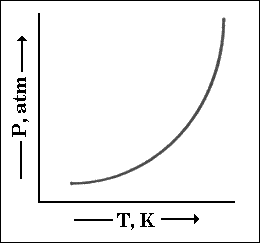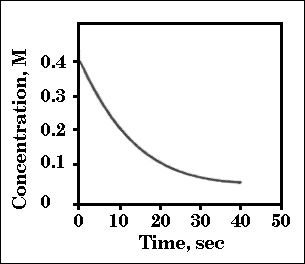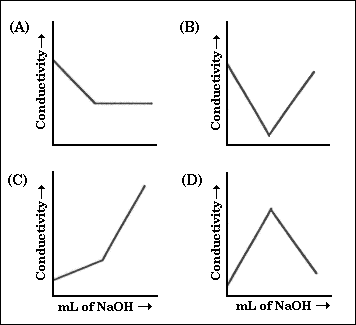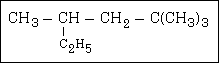MainTutorialsOrganic ChemistryPractice TestsOnline QuizzesReference ToolsU.S. National Chemistry Olympiad: 1996 National Test

Go to Answers

1. What is the formula for the basic anhydride of Ba(OH)2?

(A) Ba2O
(B) BaO
(C) BaO2
(D) Ba

2. Which element exhibits both +1 and +3 oxidation numbers in its compounds?

(A) B
(B) Be
(C) Sn
(D) Ti

3. Which element is obtained commercially by electrolysis?

(A) aluminum
(B) iron
(C) oxygen
(D) sulfur

4. A 0.1 M solution of a certain cation will form a precipitate with 0.1 M solutions of all of these anions; OH�, CO32�, SO42�. Which cation fits this description?

(A) Ba2+
(B) Fe2+
(C) Mg2+
(D) Pb2+

5. Each of these solutions is added to a mixture of aqueous sodium bromide and chloroform,CHCl3. Which one will give a positive test for aqueous bromine when the solutions are vigorously mixed?

(A) NaCl solution
(B) NaI solution
(C) NaI3 solution
(D) chlorine water

6. Oxalic acid dihydrate, H2C2O4 . 2 H2O(s) is often used as a primary standard to standardize sodium hydroxide solutions. Which of these facts are reasons to choose this substance as a primary standard?

I. It is diprotic.
II. It is a stable compound that can be weighed directly in air.
III. It is available in a pure form.

(A) III only
(B) I and II only
(C) II and III only
(D) I, II, and III

7. When FeCl3 is ignited in an atmosphere of pure oxygen,this reaction takes place.

4 FeCl3(s) + 3 O2(g) ---> 2 Fe2O3(s) + 6 Cl2(g)

If 3.0 mol of FeCl3 are ignited in the presence of 2.0 mol of O2 gas, how much of which reagent is present in excess and therefore remains unreacted?

(A) 0.33 mol FeCl3 remain unreacted
(B) 0.67 mol FeCl3 remain unreacted
(C) 0.25 mol O2 remain unreacted
(D) 0.50 mol O2 remain unreacted

8. How many grams of SbF3 are needed to produce a gram of Freon-12, CCl2F2, according to the reation represented by this equation?

3 CCl4 + 2 SbF3 ---> 3 CCl2F2+ 2 SbCl3

 Substance Molar Mass SbF3 179 g mol�1 CCl2F2 121 g mol�1

(A) 0.667g
(B) 0.986g
(C) 1.50g
(D) 2.22g

9. A self-contained breathing apparatus uses potassium superoxide, KO2, to convert the carbon dioxide and water in exhaled air into oxygen, as shown by the equation.

4 KO2(s) + 2 H2O(g) + 4 CO2(s) ---> 4 KHCO3(s) + 3 O2(g)

How many molecules of oxygen gas will be produced from the 0.0468 g of carbon dioxide that is exhaled in a typical breath?

(A) 4.8 x 1020
(B) 6.4 x 1020
(C) 8.5 x 1020
(D) 1.9 x 1021

10. Magnetite, Fe3O4, can be converted into metallic iron by heating with carbon monoxide as represented by this equation.

Fe3O4(s) + 4 CO(g) ---> 3 Fe(s) + 4 CO 2(g)

How many kilograms of Fe3O4 must be processed in this way to obtain 5.00 kg of iron if the process is 85% efficient?

 Substance Molar Mass Fe3O4 232 g mol�1

(A) 6.92 kg
(B) 8.15 kg
(C) 20.8 kg
(D) 24.4 kg

11. What is the molality of an aqueous sucrose solution that is 25.0% sucrose, C12H22O11 by mass?

 Substance Molar Mass C12H22O11 342 g mol�1

(A) 0.585 m
(B) 0.731 m
(C) 0.975 m
(D) 1.17 m

12. A student finds that 31.26 mL of a 0.165 M solution of barium hydroxide, Ba(OH)2, solution is required to just neutralize 25.00 mL of a citric acid, H3C6H5O7, solution. What is the concentration of the H3C6H5O7 solution?

(A) 0.413 M
(B) 0.309 M
(C) 0.206 M
(D) 0.138 M

13. Which Group III element is expected to have physical and chemical properties that are the least similar to the other elements in that family?

(A) B
(B) Al
(C) Ga
(D) Tl

14. A pure gas that is 14.4% hydrogen and 85.6% carbon by mass has a density of 2.5 g L�1 at 0 �C and 1 atm presure. What is the molecular formula of the gas?

(A) CH2
(B) C2H4
(C) C4H8
(D) C6H12

15. The vapor pressure of most substances increase with temperature as depicted by this curve. Which way of plotting these data is expected to give a straight line?(A) 1/P versus T
(B) P versus 1/T
(C) In P versus T
(D) In P versus 1/T

16. If 0.10 mL of liquid water are introduced into a 1.0 L flask at 25 �C, how many moles of water are in the vapor phase when equilibrium is established?

 Vapor Pressure, 25 �C H2O 23.8 mmHg

(A) 1.28 x 10�3
(B) 5.56 x 10�3
(C) 1.53 x 10�2
(D) 4.46 x 10�2

17. Sodium chloride, NaCl, usually crystallizes in a face-centered cubic lattice. How many Cl� ions are in contact with any single Na+ ion?

(A) 4
(B) 6
(C) 8
(D) 12

18. When solid NH4NO3 is dissolved in water at 25 �C, the temperature of the solution decreases. What is true about the signs of DH and DS for this process?

 DH DS (A) - + (B) - - (C) + + (D) + -

19. If the equilibrium constant for a reaction is very small (for example, 1 x 10�20), what would the value of DG be expected to be?

(A) a small positive number
(B) a large positive number
(C) a small negative number
(D) a large negative number

20. Use the bond energies in the table to determine DH for the formation of hydrazine, N2H4, from nitrogen and hydrogen according to this equation:

N2(g) + 2 H2(g) ---> N2H4(g)

 Bond Energies N-N 159 kJ mol�1 N=N 418 kJ mol�1 N {triple bond} N 941 kJ mol�1 H-H 436 kJ mol�1 H-N 389 kJ mol�1

(A) DH = 711 kJ
(B) DH = - 98 kJ
(C) DH = + 98 kJ
(D) DH = + 711 kJ

21. Use the given heats of formation to calculate the enthalpy change for this reaction:

B2O3(s) + 3 COCl2(g) ---> 2 BCl3(g) + 3 CO2(g)

 Enthalpy of Formation Data, kJ mol�1 B2O3(s) - 1272.8 COCl2(g) - 218.8 BCl3(g) - 403.8 CO2(g) - 393.5

(A) 694.3 kJ
(B) 354.9 kJ
(C) -58.9 kJ
(D) -3917.3 kJ

22. For which of these processes is the value of DS negative?

I. Sugar is dissolved in water.
II. Steam condenses on a surface.
III. CaCO3 is decomposed into CaO and CO2.

(A) I only
(B) II only
(C) I and III only
(D) II and III only

23. What is the expected value of DG� for this reaction? (E� for the reaction = 0.46 V)

Cu(s) + 2 Ag+(aq) ---> Cu2+(aq) + 2 Ag(s)

(A) -89 kJ
(B) -44 kJ
(C) -22kJ
(D) 15kJ

24. What is the change in internal energy, DE, for a system that does 70 joules of work as it absorbs 45 joules of heat?

(A) 115 J
(B) 25 J
(C) -25 J
(D) -115 J

25. What are the units of a second-order rate constant?

(A) sec�1
(B) mol L�1 sec�1
(C) mol2 L�2 sec�1
(D) L mol�1 sec�1

26. The half life for the radioactive decay of 32P is 14.3 days. How many days would be required for a sample of a radiopharmaceutical containing 32P to decrease to 20% of its initial activity?

(A) 33.2 d
(B) 61.8 d
(C) 71.5 d
(D) 286 d

27. The rates of many chemical reactions double for a ten degree rise in temperature. Which of these factors does not contribute to this change in rate with increasing temperature?

(A) the average kinetic energy of the reactant species.
(B) the number of collisions in a given time.
(C) the number of very energetic species.
(D) the activation energy.

28. The bromination of acetone that occurs in acid solution is represented by this question.

CH3COCH3(aq) + Br2(aq) ---> CH3COCH2Br(aq) + H+(aq) + Br�(aq)

These kinetic data were obtained for given reaction concentrations.

 Initial Concentrations, M Initial Rate, disappearance of Br2, M s�1 [CH3COCH3] [Br2] [H+] 0.30 0.050 0.050 5.7 x 10�5 0.30 0.10 0.050 5.7 x 10�5 0.30 0.10 0.10 1.2 x 10�4 0.40 0.050 0.20 3.1 x 10�4

Based on these data, what is the rate equation?

(A) Rate = k[CH3COCH3][Br2][H+]
(B) Rate = k[CH3COCH3][H+]
(C) Rate = k[CH3COCH3][Br2]
(D) Rate = k[CH3COCH3][Br2][H+]2

29. What is the initial rate of the reaction (in mol L�1 sec�1) depicted by this graph?(A) 0.02
(B) 0.01
(C) 0.008
(D) 0.005

30.

2 SO2(g) + O 2(g) <===> 2 SO3(g)

Given that the equilibrium constant for the reaction above has a value of 278 at a particular temperature, what is the value of the equilibrium constant for the following reaction at the same temperature?

SO3(g) <===> SO2(g) + 1/2 O2(g)

(A) 1.3 x 10�5
(B) 1.8 x 10�3
(C) 3.6 x 10�3
(D) 6.0 x 10�2

31.

Cl2(aq) + H2S(aq) ---> S(s) + 2 H+(aq) + 2 Cl�(aq)

The rate equation for this reaction is: rate = k[Cl2][H2S]

Which of these mechanisms is (are) consistent with this rate equation?

 I. Cl2 + H2S ---> H+ + Cl� + Cl+ + HS� (slow) Cl+ + HS� ---> H+ + Cl� + S (fast) II. H2S <===> H+ + HS� (fast equilibrium) Cl2 + HS� ---> 2Cl� + H+ + S (slow)

(A) I only
(B) II only
(C) Both I and II
(D) Neither I or II

32. When a sample of NO2 is placed in a container, this equilibrium is rapidly established.

2 NO2(g) <===> N2O4(g)

If this equilibrium mixture is a darker color at high temperatures and at low pressures, which of these statements about the reaction is true?

(A) The reaction is exothermic and NO2 is darker in color than N2O4.
(B) The reaction is exothermic and N2O4 is darker in color than NO2
(C) The reaction is endothermic and NO2 is darker in color than N2O4.
(D) The reaction is endothermic and N2O4 is darker in color than NO2.

33. When the acids; H2Se, HBr, and HI, are arranged in order of increasing strength (weakest acid first), which is the correct order?

(A) H2Se < HBr < HI
(B) HBr < HI < H2Se
(C) HBr < H2Se < HI
(D) HI < H2Se < HBr

34. Which statement is true about the relationship between Br�nsted-Lowry (B-L) bases and Lewis bases?

(A) A Lewis base must be a B-L base but a B-L base need not be a Lewis base.
(B) A B-L base must be a Lewis base but a Lewis base need not be a B-L base.
(C) B-L bases and Lewis bases are interchangeable.
(D) There is no relationship between Lewis and B-L bases.

35. A solution of 2.0 M formic acid (HCOOH) is 0.95% ionized. What is the Ka of formic acid?

(A) 1.9 x 10�2
(B) 1.8 x 10�4
(C) 9.0 x 10�5
(D) 4.5 x 10�5

36. If 0.1 mol of a salt is added to 1.0 L of water, which of these salts is expected to produce the most acidic solution?

(A) NaC2H3O2
(B) NH4NO3
(C) CuSO4
(D) AlCl3

37. The pKa values for several acid-base indicators are given in the table. Which indicator should be used in the titration of a weak base with a strong acid?

 Indicator, pKa 2,4-dintrophenol 3.5 bromthymol blue 7.0 cresol red 8.0 alizarin yellow R 11.0

(A) 2,4-dintrophenol
(B) bromthymol blue
(C) cresol red
(D) alizarin yellow R

38. Which graph best represents the electrical conductivity behavior that occurs when an aqueous solution of acetic acid, HC2H3O2, is titrated with an aqueous solution of sodium hydroxide, NaOH?39. When this oxidation-reduction equation is correctly balanced, what is the mole ratio of reducing agent to oxidizing agent?

MnO4� + Sn2+ + H+ ---> Mn2+ + Sn4+ + H2O

(A) 1:1
(B) 2:1
(C) 4:1
(D) 5:2

40. The solubility of solid silver chromate, Ag2CrO4, is determined in three solvents.

 Solubility Product, Ksp Ag2CrO4 9 x 10�12

I. pure water
II. 0.1 M AgNO3
III. 0.1 M Na2CrO4

Predict the relative solubility of Ag2CrO4 in the three solvents.

(A) I = II = III
(B) I < II < III
(C) II = III < I
(D) II < III < I

41. Which combination of reactants will produce the greatest voltage based on these standard electrode potentials?

 Standard Reduction Potentials E� Cu+(aq) + e� ---> Cu(s) + 0.52 V Sn4+(aq) + 2e� ---> Sn2+(aq) + 0.15 V Cr3+(aq) + e� ---> Cr2+(aq) - 0.41 V

(A) Cu+ and Sn2+
(B) Cu+ and Cr2+
(C) Cu and Sn4+
(D) Sn4+ and Cr2+

42. The reduction of oxygen gas in 1 M aqueous acid is represented by this equation: (E� = 1.23 V)

O2(g) + 4H+(aq) + 4e� ---> 2H2O(l)

The potential for this reaction at physiological pH, where [H+] = 1 x 10�7 and the partial pressure of O2 is 1 atm, will be closest to which value?

(A) 0.18 V
(B) 0.41 V
(C) 0.82 V
(D) 1.64 V

43. What products are formed during the electrolysis of a concentrated aqueous solution of sodium chloride?

I. Cl2(g)
II. NaOH(aq)
III. H2(g)

(A) I only
(B) I and II only
(C) I and III only
(D) I, II, and III

44. A current of 5.00 A is passed through an aqueous solution of chromium(III) nitrate for 30.0 min. How many grams of chromium metal will be deposited at the cathode?

(A) 0.027 g
(B) 1.62 g
(C) 4.85 g
(D) 6.33 g

45. The hydrogen line spectrum provides evidence for the

(A) Heisenberg Uncertainly Principle
(B) wavelike properties of light
(C) diatomic nature of H2
(D) quantized nature of atomic energy states

46. What is the number of unpaired electrons in a manganese atom (Z = 25) in its lowest energy states?

(A) 1
(B) 3
(C) 5
(D) 7

47. Ions with the electronic structure 1s2 2s2 2p6 3s2 3p6 would not be present in which aqueous solution?

(A) NaF(aq)
(B) NaCl(aq)
(C) KBr(aq)
(D) CaI2(aq)

48. An element with the electron configuration [Xe]4f14 5d7 6s2, is

(A) an alkaline earth element
(B) a transition element
(C) an inert gas
(D) a rare earth

49. The neutron/proton ratio in an isotope can be increased by the emission of

(A) an electron
(B) a neutron
(C) a gamma ray
(D) a positron

50. The bonds in ozone, O3, are best represented as

(A) distinct single and double bonds
(B) a single and a double bond that switch positions rapidly
(C) something between a single and a double bond
(D) two double bonds

51. Which pair of substances will have the most similar geometry?

(A) SO3 and SO32
(B) SO3 and CO32
(C) SO3 and SO42
(D) SO42� and CO32

52. What hybridization is expected for ClF3?

(A) sp3
(B) dsp2
(C) dsp3
(D) d2sp3

53. Which bond properties are consistent with one another?

 Bond Order Bond Length Vibrational Frequency (A) higher shorter higher (B) higher longer lower (C) lower shorter lower (D) lower longer higher

54. Which hydrogen halide has the lowest boiling point?

(A) HF
(B) HCl
(C) HBr
(D) HI

55. Which substance has a dipole moment?

(A) CCl4
(B) CH2Cl2
(C) C2Cl2
(D) C2Cl4

56. What is the correct name for this compound?(A) 2-ethyl-4, 4-dimethylpentane
(B) 2-methyl-4-t-butylbutane
(C) 2,2,4-trimethylhexane
(D) 1-t-butyl-2-methylbutane

57. How many different structures exist for C2H2Cl2?

(A) 1
(B) 2
(C) 3
(D) 4

58. When ethanol is heated with sulfuric acid, the major product is

(A) ethane
(B) ethene
(C) ethyne
(D) ethyl sulfate

59. Many alcohols can be oxidized to aldehydes, and aldehydes can be oxidized to

(A) carboxylic acids
(B) esters
(C) ethers
(D) ketones

60. Which statement about polymers is not true?

(A) They are macromolecules
(B) They have simple repeating units
(C) They have very high molecular masses
(D) They are all made by chemistsnews | about us | contact us tutorials index | organic chemistry | practice tests | online quizzes | reference tools site copyright (c) 2002-2013 Learn ChemSubscribe to our low volume newsletter to receive up-to-date information about the CHEM SITE